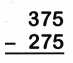# McGraw Hill Math Grade 2 Chapter 5 Lesson 4 Answer Key Subtracting Three-Digit Numbers

Practice questions available in McGraw Hill Math Grade 2 Answer Key PDF Chapter 5 Lesson 4 Subtracting Three-Digit Numbers will engage students and is a great way of informal assessment.

## McGraw-Hill Math Grade 2 Answer Key Chapter 5 Lesson 4 Subtracting Three-Digit Numbers

Subtract

Write the difference. Regroup if you need to.

Question 1.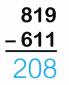Question 2.Explanation:
The difference of 780 and 331 is 449.

Question 3.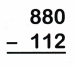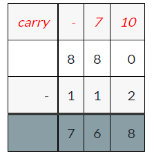Explanation:
The difference of 880 and 112 is 768.

Question 4.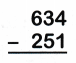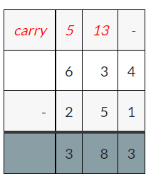Explanation:
The difference of 634 and 251 is 383.

Question 5.Explanation:
The difference of 458 and 399 is 59.

Question 6.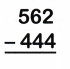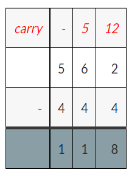Explanation:
The difference of 562 and 444 is 118.

Question 7.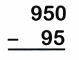Explanation:
The difference of 950 and 95 is 855.

Question 8.